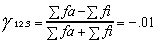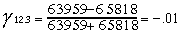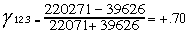previous lesson

Partial gamma

ORDINAL DESCRIPTIVE MULTIVARIATE DISTRIBUTION

Partial gamma:Where: fa 12.3 = fa between variable #1 and variable #2 for a subtable of variable #3.

fi 12.3 = fi between variable # 1 and #2 for a subtable of variable #3.

Note: Compute fa and fi same as for gamma.
Variable #3 is always the variable held constant.
Variable #3 is assumed to be nominal; variables #1 and #2 must be at least ordinal.

Interpretation of partial gamma: just like regular gamma, but add the information from the 3rd variable.

Example:

 College Education High School Ed. Less than High School Ed. Income Income Income Age Hi Lo Hi Lo Hi Lo 45+ 104 36 45+ 159 179 45+ 54 335 45- 163 46 45- 290 327 45- 24 133

Q: What is the relationship between age and income, with education held constant?
A: First, check level of measurements: age and income (variables #1 and #2) are both ordinal in this case, and education (variable #3) is nominal.

 VARIABLE #3 EDUCATION fa12.3 fi12.3 COLLEGE 104(46)= 4,784 36(163)= 5,868 HIGH SCHOOL 159(327)=51,993 179(290)=51,910 LESS THAN HIGH SCHOOL 54(133)= 7,182 335(24)= 8,040 fa12.3= 63,959 fi12.3= 65,818Interpretation: A very small negative association between age and income with education held constant. 1% more disagreement than agreement in rank order between age and income with education held constant.

Q2: What is the relationship between income and education with age held constant?
A2: First, make new tables to get the data in the form needed.

 45+ Under 45 Income Income Education Hi Lo Education Hi Lo C 104 36 C 163 46 HS 159 179 HS 290 327

 VARIABLE #3 AGE fa12.3 fi12.3 45+ 104(179 + 335) + 159(335) = 106,721 36(159 + 54) + 179(54)= 17,334 45 163(327 + 133) + 290(133)= 113,550 46(290 + 24) + 327(24) = 22,292 fa12.3 = 220,271 fi12.3 = 39,626Interpretation: " There is a moderately high positive association between income and education with age held constant." Or "There is 70% more agreement than disagreement in rank order between income and education with age held constant".